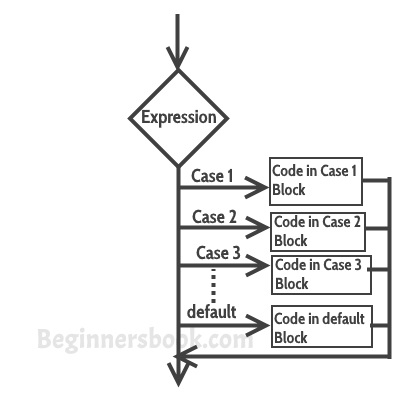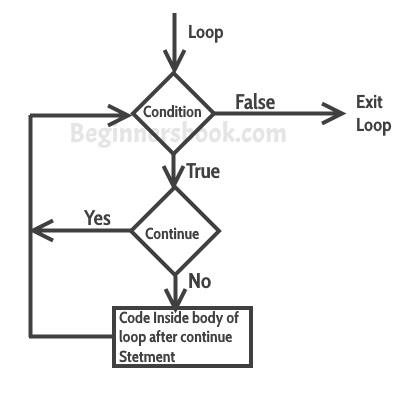# C Tutorial

## Program to print Fibonacci Series using Recursion

Program to print Fibonacci Series using Recursion A Fibonacci series is defined as a series in which each number is

## Program to find Factorial of a Number using Recursion

Program to find Factorial of a Number using Recursion #include<stdio.h> // declaring the function int fact(int); int main() { printf("\n\n\t\tEduguru

## C Program for Adding Two Numbers Using Recursion

C Program for Adding Two Numbers Using Recursion #include<stdio.h> int y; /* Function to add two numbers and return the

## C Program find whether a Number is Prime Or Composite using Recursion

C Program find whether a Number is Prime Or Composite using Recursion Prime Number: A number that is only divisible by

## C program to print digital clock with current time

#include <stdio.h> #include <time.h>   int main() {     time_t s, val = 1;     struct tm* current_time;        s = time(NULL);

## Pointers in C Programming

A pointer is a variable that stores the address of another variable. Unlike other variables that hold values of a

## Structure in C programming

Structure is a group of variables of different data types represented by a single name. Lets say we need to

## Function call by reference in C Programming

Before we discuss function call by reference, lets understand the terminologies that we will use while explaining this: Actual parameters:

## Functions in C Programming

A function is a block of statements that performs a specific task. Suppose you are building an application in C

## Strings and String functions in cString is an array of characters. In this post, we learn how to declare strings, how to work with strings

## Two dimensional (2D) arrays in C programmingAn array of arrays is known as 2D array. The two dimensional (2D) array in C programming is also known

## Arrays in C programmingAn array is a group (or collection) of same data types. For example an int array holds the elements of

## goto statement in c programmingThe goto statement is rarely used because it makes program confusing, less readable and complex. Also, when this is used,The switch case statement is used when we have multiple options and we need to perform a different task forThe continue statement is used inside loops. When a continue statement is encountered inside a loop, control jumps to the